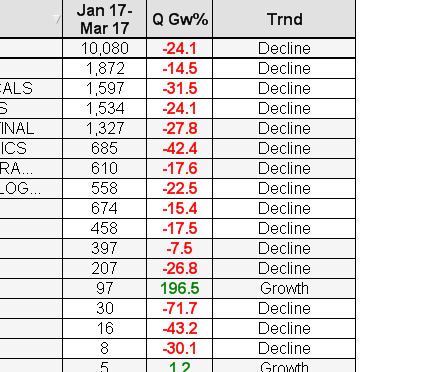# New to QlikView

Discussion board where members can get started with QlikView.

Announcements
QlikWorld, June 24-25, 2020. Free virtual event for Data Integration and Data Analytics gurus. Register Now
cancel
Showing results for
Did you mean:
HighlightedContributor III

## Need help in Pivot tableHi All,

I have create pivot table seller name,quarter as dimension and sales amount as expression.

How can i create pivot table with column %QoQ and Trend as shown in the picture.

4 Replies
HighlightedMaster III

## Re: Need help in Pivot table

What is your %QoQ Logic based on logic you can create that %QoQ column and after creation of %QoQ simply create new column like

if( %QoQ  < 0  , 'Decline' , 'Growth')

HighlightedContributor III

## Re: Need help in Pivot table

Logic for-  %QoQ is (Current quarter-Previous quarter)/Previous quarter. If i add column in pivot it will show for all expression. I need end of the pivot.

HighlightedMaster III

## Re: Need help in Pivot table

You want like this?Try below expression

(( ((SUM({\$<FinancialYear=,Quarter=,MonthName=,FiscalYear={\$(=max(FiscalYear))}
,
[Posting Date]={">=\$(=QuarterStart(max({\$<FiscalYear={\$(=max(FiscalYear))}>}[Posting Date])))<=\$(=max({\$<FiscalYear={\$(=max(FiscalYear))}>}[Posting Date]))"}
>}
[Sales Amount])/100000))-

((
Sum({\$<FinancialYear=,Quarter=,MonthName=,FiscalYear={\$(=max(FiscalYear)-1)}
,
>}
[Sales Amount])

)/100000))/

fabs(((Sum({\$<FinancialYear=,Quarter=,MonthName=,FiscalYear={\$(=max(FiscalYear)-1)}
,
>}
[Sales Amount])

)/100000))
)*100

Or Please share some sample app

HighlightedContributor III

Yes.. correct.